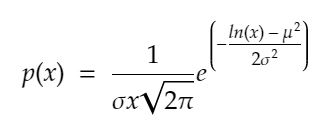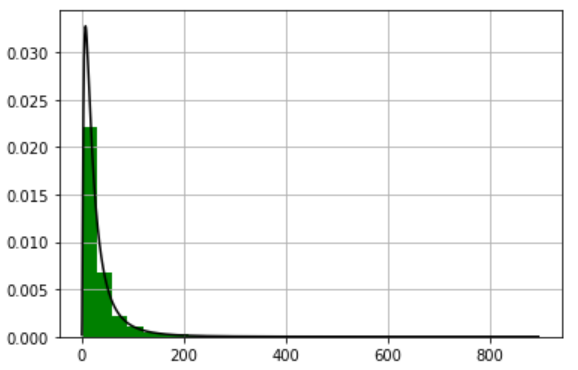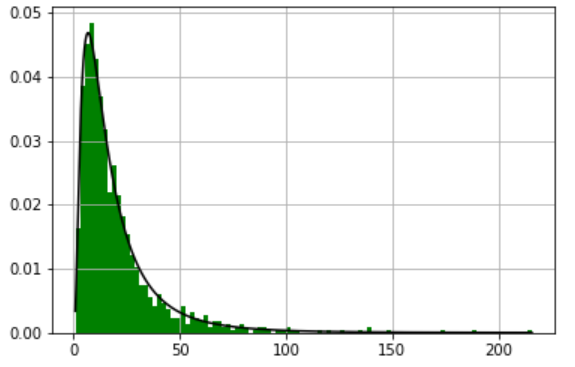# How to generate random numbers from a log-normal distribution in Python ?

• Last Updated : 07 Apr, 2021

A continuous probability distribution of a random variable whose logarithm is usually distributed is known as a log-normal (or lognormal) distribution in probability theory.

A variable x is said to follow a log-normal distribution if and only if the log(x) follows a normal distribution. The PDF is defined as follows.

Attention geek! Strengthen your foundations with the Python Programming Foundation Course and learn the basics.

To begin with, your interview preparations Enhance your Data Structures concepts with the Python DS Course. And to begin with your Machine Learning Journey, join the Machine Learning - Basic Level CourseProbability Density function Log-normal

Where mu is the population mean & sigma is the standard deviation of the log-normal distribution of a variable. Just like normal distribution which is a manifestation of summation of a large number of Independent and identically distributed random variables, lognormal is the result of multiplying a large number of Independent and identically distributed random variables. Generating a random number from a log-normal distribution is very easy with help of the NumPy library.

Syntax:

numpy.random.lognormal(mean=0.0, sigma=1.0, size=None)

Parameter:

• mean: It takes the mean value for the underlying normal distribution.
• sigma: It takes only non-negative values for the standard deviation for the underlying normal distribution
• size : It takes either a int or a tuple of given shape. If a single value is passed it returns a single integer as result. If a tuple then it returns a 2D matrix of values from log-normal distribution.

Returns: Drawn samples from the parameterized log-normal distribution(nd Array or a scalar).

The below example depicts how to generate random numbers from a log-normal distribution:

## Python3

 `# import modules``import` `numpy as np``import` `matplotlib.pyplot as plt`` ` `# mean and standard deviation``mu, sigma ``=` `3.``, ``1.`  `s ``=` `np.random.lognormal(mu, sigma, ``10000``)`` ` `# depict illustration``count, bins, ignored ``=` `plt.hist(s, ``30``,``                                ``density``=``True``, ``                                ``color``=``'green'``)``x ``=` `np.linspace(``min``(bins),``                ``max``(bins), ``10000``)`` ` `pdf ``=` `(np.exp(``-``(np.log(x) ``-` `mu)``*``*``2` `/` `(``2` `*` `sigma``*``*``2``))``       ``/` `(x ``*` `sigma ``*` `np.sqrt(``2` `*` `np.pi)))`` ` `# assign other attributes``plt.plot(x, pdf, color``=``'black'``)``plt.grid()``plt.show()`

Output:Let’s prove that log-Normal is a product of independent and identical distributions of a random variable using python. In the program below we are generating 1000 points randomly from a normal distribution and then taking the product of them and finally plotting it to get a log-normal distribution.

## Python3

 `# Importing required modules``import` `numpy as np``import` `matplotlib.pyplot as plt`` ` `b ``=` `[]`` ` `# Generating 1000 points from normal distribution.``for` `i ``in` `range``(``1000``):``    ``a ``=` `12.` `+` `np.random.standard_normal(``100``)``    ``b.append(np.product(a))`` ` `# Making all negative values  into positives``b ``=` `np.array(b) ``/` `np.``min``(b)``count, bins, ignored ``=` `plt.hist(b, ``100``, ``                                ``density``=``True``, ``                                ``color``=``'green'``)`` ` `sigma ``=` `np.std(np.log(b))``mu ``=` `np.mean(np.log(b))`` ` `# Plotting the graph.``x ``=` `np.linspace(``min``(bins), ``max``(bins), ``10000``)``pdf ``=` `(np.exp(``-``(np.log(x) ``-` `mu)``*``*``2` `/` `(``2` `*` `sigma``*``*``2``))``       ``/` `(x ``*` `sigma ``*` `np.sqrt(``2` `*` `np.pi)))`` ` `plt.plot(x, pdf,color``=``'black'``)``plt.grid()``plt.show()`

Output:My Personal Notes arrow_drop_up